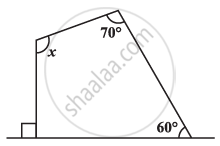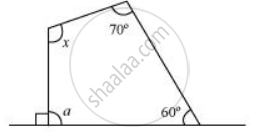# Find the Angle Measure X in the Given Figure - 2 - Mathematics

Find the angle measure x in the given Figure#### SolutionFrom the figure, it can be concluded that,

90º + a = 180º (Linear pair)

a = 180º − 90º = 90º

Sum of the measures of all interior angles of a quadrilateral is 360º. Therefore, in the given quadrilateral,

60° + 70° + x + 90° = 360°

220° + x = 360°

x = 140°

Concept: Angle Sum Property of a Quadrilateral
Is there an error in this question or solution?

#### APPEARS IN

NCERT Class 8 Maths